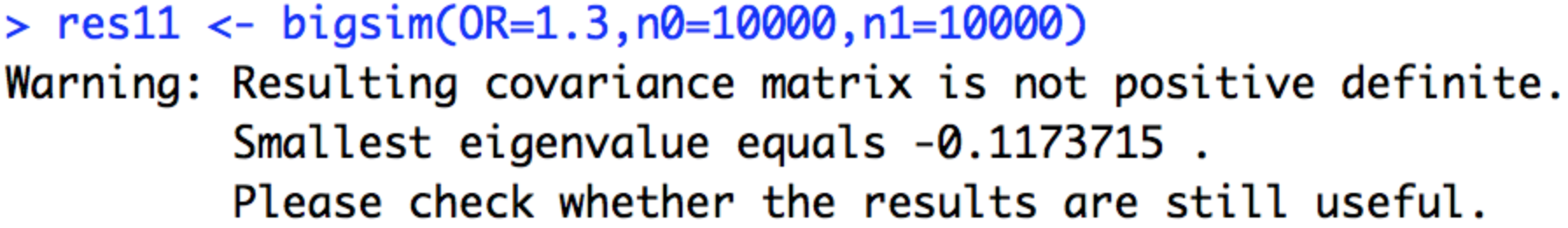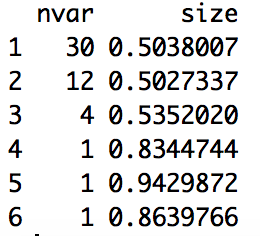### Estimating coverage using samples from the same distribution

#### 1. Dirichlet method:

Method:

1. Use the posterior probability vector (pp) to define the dirichlet distribution, and take many samples from it. $p^* \sim dirichlet(pp)$

2. Sort each sample into descending order.

3. Cumulatively sum the elements in each sample until the pre-defined threshold is reached.

4. Estimate $$p(causal.in.set)$$ by $$\sum_i PP_i \times I(i \in set^*)$$. I.E. go back to the original ordered posterior probabilities, and sum the first $$w$$ elements, defined by how many elements were needed to reach the threshold in the sample.

Problem:

• PP samples taken from the Dirichlet distribution are too variable.

• Even for the OR=1 scenario, we had some samples with one SNP holding >0.9 the posterior probability. For these samples, only 1 variant ($$w=1$$) will be in the credible set, so when we go back to the original system and sum posteior probabilities for the first $$w$$ variants to estimate coverage, we get very very low coverage.

#### 2. Investigate variability in PP samples

A function, bigsim, was written to simulate posterior probability samples from the same distribution.

# function to generate pp samples
library(mvtnorm)
library(bindata)
library(simGWAS)

bigsim <- function(OR=1.2,n0=10000,n1=10000,nsnps=100,nhaps=1000,LD1=0.3,LD2=0.25) {

maf <- runif(nsnps,0.05,0.4) # doesnt run if >0.4

R<-diag(1,nsnps)
R[cbind(1:(nsnps-1),2:nsnps)] <- LD1
R[cbind(2:nsnps,1:(nsnps-1))] <- LD1
R[cbind(1:(nsnps-2),3:nsnps)] <- LD2
R[cbind(3:nsnps,1:(nsnps-2))] <- LD2
haps=rmvbin(nhaps, maf, bincorr=R)
snps <- colnames(haps) <- paste0("s",1:nsnps)

CV=sample(snps[which(colMeans(haps)>0.1)],1)

freq=haps+1
freq=as.data.frame(freq)
snps <- colnames(freq) <- paste0("s",1:nsnps)
freq$Probability <- 1/nrow(freq) freq g1 <- OR zsim <- simulated_z_score(N0=n0, # number of controls N1=n1, # number of cases snps=snps, # column names in freq of SNPs for which Z scores should be generated W=CV, # causal variants, subset of snps gamma.W=log(g1), # log odds ratios freq=freq, # reference haplotypes nrep=1000) psim <- pnorm(abs(zsim),lower.tail=FALSE)*2 ppsim <- lapply(1:nrow(zsim), function(i) { tmp <- coloc:::approx.bf.p(p = psim[i,], f=colMeans(haps), type = "cc", N=n0+n1, s=n1/(n0+n1))[,"lABF"] p1 <- 1e-04 # hard coded - bad code - can fix later nsnps <- length(tmp) prior <- c(1-nsnps*p1,rep(p1,nsnps)) tmp <- c(1,tmp) # add on extra for null model my.denom <- coloc:::logsum(tmp + prior) exp(tmp - my.denom)[-1] }) %>% do.call("rbind",.) # simulated posterior probabilities return(list(ppsim=ppsim,zsim=zsim,psim=psim,CV=as.numeric(sub("s","",CV)))) } res <- bigsim() ppsim <- res$ppsim # 1000*nsnps
zsim <- res$zsim CV <- res$CV # causal variant

NOTE, I am getting this error - need to check results still ok.### 3. Obtaining credible sets

The general method to obtain a credible set is given below. The estimate of coverage (‘reported coverage’) is given by the sum of the posterior probabilities of the variants in the credible set.

pp <- lowest[]$PP # choose a random vector of posterior probabilities or <- order(pp, decreasing=TRUE) # find the indices from ordering pp.ord <- pp[or] # vector of ordered posterior probabilities nvar <- which(cumsum(pp[or])>0.5) # how many variants needed to reach thr sum(pp[or[1:nvar]]) # sum the first w variants in the ordered set This standard estimate of coverage is called ‘reported coverage’ from now onwards We hope to use PP samples to get a more accurate estimate of coverage. Chris’s idea was to form credible sets from the samples and note how many variants in each set (w) and then go back to the original system and sum these first w variants. Do this for each sample and average. The following function, h generates credible sets for each sample. The output is a list of the number of variants and the size of each credible set. h=function(i) { p=ppsim[i,] # choose a sample (row) from y o=order(p,decreasing=TRUE) # indices from ordering sample nvar=which(cumsum(p[o])>0.5) size=sum(p[o[1:nvar]]) result <- list(nvar=nvar,size=size) return(result) } cs <- as.data.frame(t(sapply(1:nrow(ppsim), h))) cs$nvar <- unlist(cs$nvar) cs$size <- unlist(cs$size) head(cs)We can already see the variability in the samples, whereby the first sample seems to have fairly low entropy (fair few variants needed to reach the threshold) and samples 4-6 seem to have very high entropy where 1 variant holds >80% of the posterior probability. Note that this was for an OR=1.1 scenario. ### Method 1. Use the bigsim function to generate many samples (n). 2. For each of these, form a credible set using the normal method and note how many variants in each of the credible sets ($$w_1,...,w_n$$). 3. For each $$w_1,...,w_n$$, go back to the original system and sum these elements. 4. Average the results to get an estimate of true coverage. Code: ## Reported coverage res <- bigsim() # specify OR in here ppsim <- res$ppsim
pp <- colMeans(ppsim) # true pp
or <- order(pp, decreasing=TRUE)
pp.ord <- pp[or]
nvar <- which(cumsum(pp[or])>0.5)
sum(pp[or[1:nvar]]) # reported coverage using normal method

## Using simulations to estimate true coverage
f=function(i) {
p=ppsim[i,] # choose a sample (row) from y
o=order(p,decreasing=TRUE) # indices from ordering sample
w=which(cumsum(p[o])>0.5)  # how many variants needed to reach thr
sum(pp[o[1:w]])
}
mean(sapply(1:nrow(ppsim), f))

### Results

• The ‘cumsum’ column gives the reported coverage (the sum of the posterior probabilities of the variants in the credible set for the true pp, which here is the colMeans of ppsim).

• The ‘using simulations’ column uses the simulations and the function f Chris wrote (above) to provide an estimate of the true coverage.

• The bias column is $$cumsum-using simulations$$. A negative bias value implies the reported coverage value (using the standard method) is too high, and a positive bias value implies that this value is too low.

• From Hope’s research, we expect negative bias values for low OR (undercoverage), approximately 0 bias values for medium OR and positive bias values for high OR (overcoverage).

#### 1. N0=N1=10000, 1000 simulations

Cumsum Using simulations Bias
1) OR=1 0.502 0.216 -0.289
2) OR=1 0.507 0.208 -0.299
3) OR=1 0.504 0.208 -0.296
4) OR=1.05 0.504 0.228 -0.276
5) OR=1.05 0.501 0.246 -0.255
6) OR=1.05 0.507 0.204 -0.303
7) OR=1.1 0.648 0.630 -0.018
8) OR=1.1 0.502 0.389 -0.113
9) OR=1.1 0.627 0.618 -0.009
10) OR=1.15 0.993 0.992 -0.001
11) OR=1.15 0.916 0.912 -0.004
12) OR=1.15 0.998 0.998 0
13) OR=1.2 0.999 0.999 0
14) OR=1.2 0.999 0.999 0
15) OR=1.2 0.999 0.999 0

#### 2. N0=N1=50000 (to try and cut down variability), 1000 simulations

Cumsum Using simulations Bias
1) OR=1 0.502 0.296 -0.206
2) OR=1 0.505 0.285 -0.220
3) OR=1 0.503 0.298 -0.205
4) OR=1.05 0.914 0.909 -0.005
5) OR=1.05 0.880 0.876 -0.004
6) OR=1.05 0.872 0.864 -0.006
7) OR=1.1 0.999 0.999 0
8) OR=1.1 0.999 0.999 0
9) OR=1.1 0.999 0.999 0
10) OR=1.15 0.999 0.999 0
11) OR=1.15 0.999 0.999 0
12) OR=1.15 0.999 0.999 0
13) OR=1.2 0.999 0.999 0
14) OR=1.2 0.999 0.999 0
15) OR=1.2 0.999 0.999 0
• Notice, since the sample size is so big, the power of the system is high. Even a tiny increase in effect size (e.g. OR=1.05) leads to high disorder posterior probability systems, with one SNP holding most of the probability.

#### 3. N0=N1=10000, 5000 simulations

Cumsum Using simulations Bias
1) OR=1 0.502 0.208 -0.294
2) OR=1 0.502 0.209 -0.293
3) OR=1 0.501 0.208 -0.293
4) OR=1.05 0.502 0.207 -0.295
5) OR=1.05 0.505 0.205 -0.3
6) OR=1.05 0.502